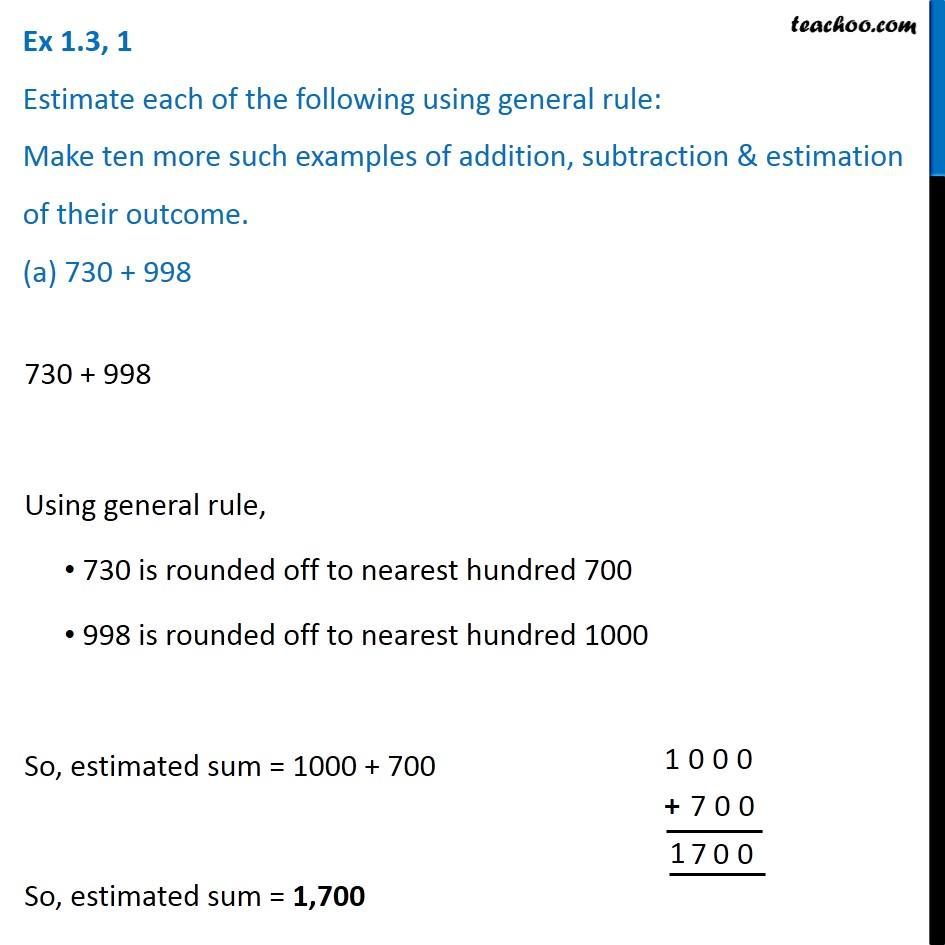1. Chapter 1 Class 6 Knowing our Numbers
2. Concept wise
3. Estimating sum and difference

Transcript

Ex 1.3, 1 Estimate each of the following using general rule: Make ten more such examples of addition, subtraction & estimation of their outcome. (a) 730 + 998 730 + 998 Using general rule, 730 is rounded off to nearest hundred 700 998 is rounded off to nearest hundred 1000 So, estimated sum = 1000 + 700 So, estimated sum = 1,700